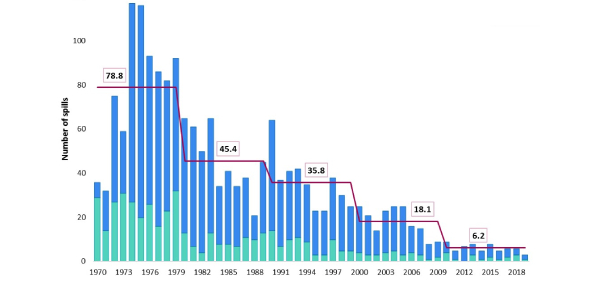# Statistics Practice Test Questions! Quiz

30 Questions | Total Attempts: 130Settings.

Related Topics
• 1.
Which of the following are types of scales used in measurement issues
• A.

Ordinal

• B.

Nominal, interval

• C.

Ratio

• D.

All of the above

• 2.
If one is concerned about exactly what a scale is measuring, then that reflects the issue of
• A.

Reliability

• B.

Validity

• C.

Internal validity

• D.

External validity

• 3.
Saying that “depression is defined here as a Beck’s Depression Inventory score of 8 or higher” is an example of
• A.

Reliability

• B.

Internal validity

• C.

Operationalization

• D.

Construct validity

• 4.
Concerns over-reactivity are greatest for ____ designs.
• A.

Observational

• B.

Survey

• C.

Experimental

• D.

Archival

• 5.
Which of the following is an example of a “construct?”
• A.

Depression

• B.

Intelligence

• C.

Anxiety

• D.

All of the above are examples

• 6.
Examining the “leftovers” of some behavior of interest is an example of:
• A.

Archival research

• B.

Naturalistic observation

• C.

Reliability

• D.

Behavioral products

• 7.
Which of the following indicates the strongest relationship?
• A.

R = -.87

• B.

R = .28

• C.

R = .69

• D.

R = -.73

• 8.
Saying that a correlation is “statistically significant” implies:
• A.

That is sufficiently strong

• B.

That the true value (rho) is unlikely zero

• C.

That the relationship is positive rather than negative

• D.

Variable variable variable the x variable causes the y variable

• 9.
The main concern over “truncated range” in correlation is that the correlation value is:
• A.

Equal to zero

• B.

Likely to be negative

• C.

Likely to be less than the true value (rho)

• D.

All of the above

• 10.
If self-esteem increases as depression decreases, this is an example of a _____ relationship.
• A.

Positive

• B.

Reliable

• C.

Valid

• D.

Negative

• 11.
If you’re comparing two groups and you suspect that members of one group experienced something outside of experimental treatment, then you would be suspecting the influence of:
• A.

Maturation

• B.

Concurrent history

• C.

Regression towards the mean

• D.

Instrumentation

• 12.
For which of the following designs would the testing effect be least likely to influence results:
• A.

Posttest only control group design

• B.

Pretest posttest control group design

• C.

Solomon Four Group design

• D.

All of the above are susceptible to testing effect

• 13.
For experimental designs, external validity tends to be dealt with via:
• A.

Random assignment of subjects to groups

• B.

Choice of design

• C.

Choice of subjects

• D.

Replication

• 14.
Subjects getting hungry and less attentive would be an example of:
• A.

Concurrent history

• B.

Instrumentation

• C.

Maturation

• D.

Testing effect

• 15.
The major threat for quasi-experimental designs is:
• A.

Selection bias

• B.

Instrumentation

• C.

Regression towards the mean

• D.

Mortality

• 16.
If one or more of your groups has unusually high or low scores, then you maybe risking the threat of:
• A.

Selection bias

• B.

Instrumentation

• C.

Regression towards the mean

• D.

Mortality

• 17.
If you have an experiment with two IVs, then this would be a _______ design.
• A.

Pretest posttest control group

• B.

Solomon Four Group

• C.

Posttest only control group

• D.

Factorial

• 18.
When the experimenter’s expectations potentially influence your results, this represents:
• A.

Demand characteristics

• B.

Experimenter bias

• C.

Reactivity

• D.

Subject error

• 19.
One acceptable solution to demand characteristics would be:
• A.

Distraction

• B.

Concealment

• C.

Deception

• D.

All of the above

• 20.
In the graph below, identify which of the following effects is present (put “yes” or “no” on each blank): IV1 _ IV2 _ IV1 X IV2 __
• 21.
If you're interested in a non-reactive design examining people's eating habits, you might consider doing a              study.
• A.

Observational

• B.

Experimental

• C.

Behavioral products

• D.

Quasi-experimental

• 22.
When we're concerned about the consistency of some test or measure, we're concerned about:
• A.

Reliability

• B.

Validity

• C.

Internal validity

• D.

External validity

• 23.
The design mean to show that change in the IV cause changes in the DV is:
• A.

Correlational

• B.

Quasi-experimental

• C.

Experimental

• D.

Observational

• 24.
Defining some variable in terms of how it is measured is:
• A.

Internal validity

• B.

Operationalization

• C.

Reliability

• D.

Validity

• 25.
Salary, is defined in terms of dollars earned per year, is an example of a            scale.
• A.

Nominal

• B.

Ordinal

• C.

Interval

• D.

Ratio

• 26.
Z-score, where 0 refers to the average score, is an example of            scale.
• A.

Nominal

• B.

Ordinal

• C.

Interval

• D.

Ratio

• 27.
Reactivity is mainly a problem for what type of design
• A.

Experimental

• B.

Quasi-experimental

• C.

Observational

• D.

All of the above

• 28.
When one variable increases while the other decreases, that is an example of a           design.
• A.

Direct

• B.

Inverse

• C.

Tolerant

• D.

Invalid

• 29.
If you do not have the full range of X or Y variables in a correlational design, then you run the risk of what problem?
• A.

Truncated range

• B.

Absolute value

• C.

Inverse design

• D.

All of the above

• 30.
Variable that do not physically exist (e.g., depression) are called:
• A.

Independent Variables

• B.

Dependent variables

• C.

Invisible variables

• D.

Constructs